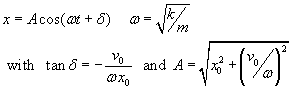### Mass on a Spring - Figuring out equation from initial conditions

 The equations used here are :Step 1) Enter the starting conditions : Mass (m) = kg Spring constant (K) = N/m Initial Position (x0) = m (R/L = +/-) Initial Velocity (v0) = m (R/L = +/-) Step 2) Update the graph values :    Based on your choices above, these values are calculated: Omega (w) = rad/sec Amplitude (A) = meters Phase angle (phi) = radians Period (T) = sec E total = Joules Things to try : Have +x0 and +v0 value Have a +x0 and a -v0 value (how does it differ) Have x0=0 and +V0 - compare to +x0/v0=0 If you have v0=0 .. how does changing the mass affect the energy? Why? If you have x0=0 .. how does changing K affect the energy, why?